# DIFFERENTIAL EQUATIONS Dr Ir Harinaldi M Eng Mechanical

• Slides: 39DIFFERENTIAL EQUATIONS Dr. Ir. Harinaldi, M. Eng Mechanical Engineering Department Faculty of Engineering University of Indonesia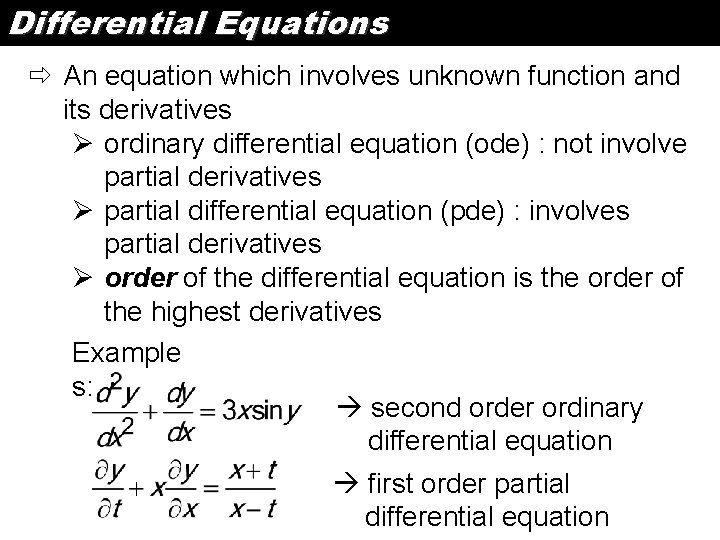Differential Equations ð An equation which involves unknown function and its derivatives Ø ordinary differential equation (ode) : not involve partial derivatives Ø partial differential equation (pde) : involves partial derivatives Ø order of the differential equation is the order of the highest derivatives Example s: second order ordinary differential equation first order partial differential equationDifferential Equations Modeling via Differential Equations Note that the set of equations is called a Model for the system. How do we build a Model? The basic steps in building a model are: Step 1: Clearly state the assumptions on which the model will be based. These assumptions should describe the relationships among the quantities to be studied. Step 2: Completely describe the parameters and variables to be used in the model. Step 3: Use the assumptions (from Step 1) to derive mathematical equations relating the parameters and variables (from Step 2).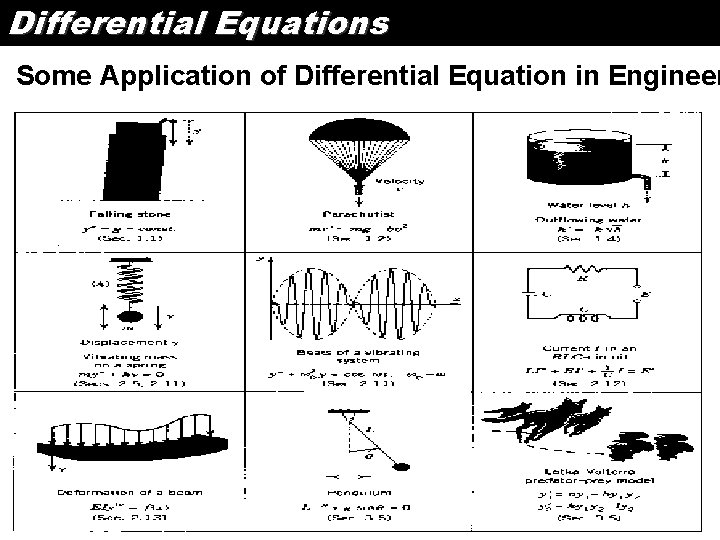Differential Equations Some Application of Differential Equation in Engineer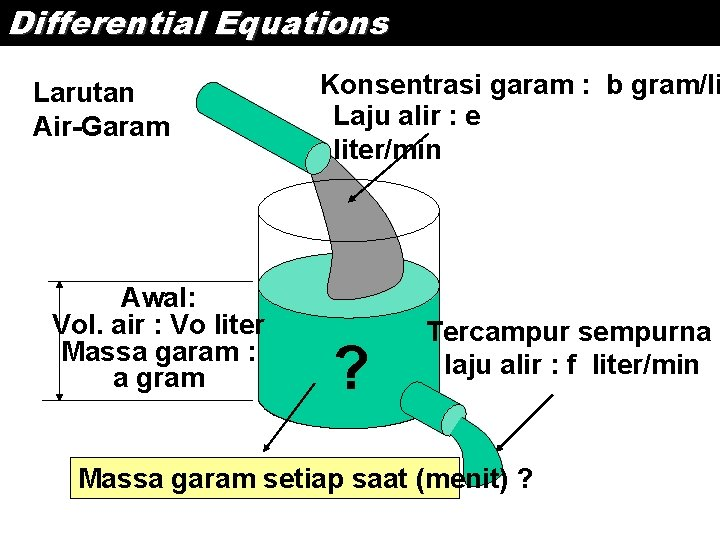Differential Equations Larutan Air-Garam Awal: Vol. air : Vo liter Massa garam : a gram Konsentrasi garam : b gram/li Laju alir : e liter/min ? Tercampur sempurna laju alir : f liter/min Massa garam setiap saat (menit) ?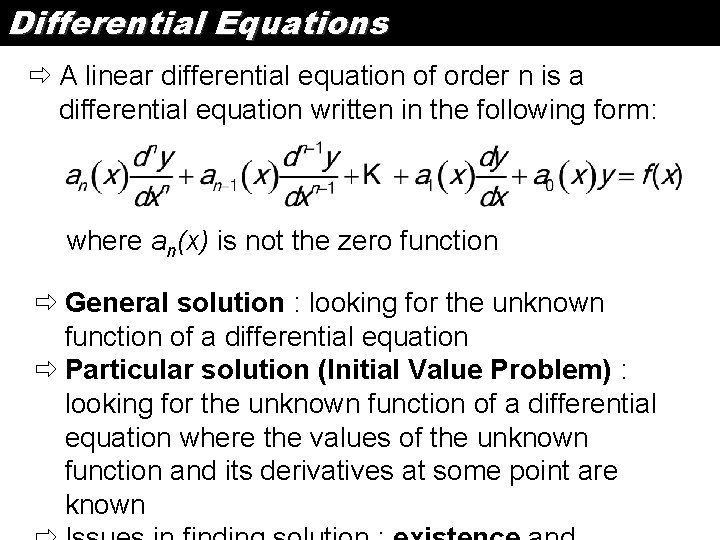Differential Equations ð A linear differential equation of order n is a differential equation written in the following form: where an(x) is not the zero function ð General solution : looking for the unknown function of a differential equation ð Particular solution (Initial Value Problem) : looking for the unknown function of a differential equation where the values of the unknown function and its derivatives at some point are known1 st Order DE - Separable Equations The differential equation M(x, y)dx + N(x, y)dy = 0 is separable if the equation can be written in the form: Solution : 1. Multiply the equation by integrating factor: 2. The variable are separated : 3. Integrating to find the solution:1 st Order DE - Separable Equations Example s: 1. Solve : Answer:1 st Order DE - Separable Equations Example s: 1. Solve : Answer:1 st Order DE - Separable Equations Example s: 2. Find the particular solution of : Answer: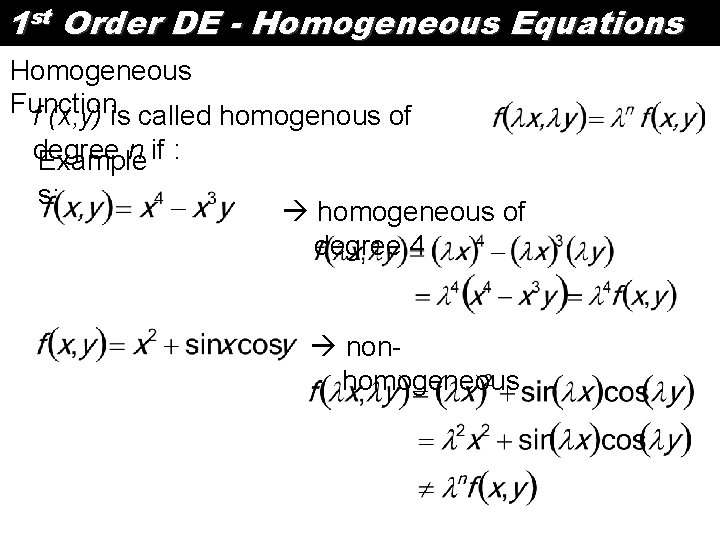1 st Order DE - Homogeneous Equations Homogeneous Function f (x, y) is called homogenous of degree n if : Example s: homogeneous of degree 4 nonhomogeneous1 st Order DE - Homogeneous Equations The differential equation M(x, y)dx + N(x, y)dy = 0 is homogeneous if M(x, y) and N(x, y) are homogeneous and of the same degree Solution : 1. Use the transformation to : 2. The equation become separable equation: 3. Use solution method for separable equation 4. After integrating, v is replaced by y/x1 st Order DE – Homogeneous Equations Example s: 1. Solve : Answer: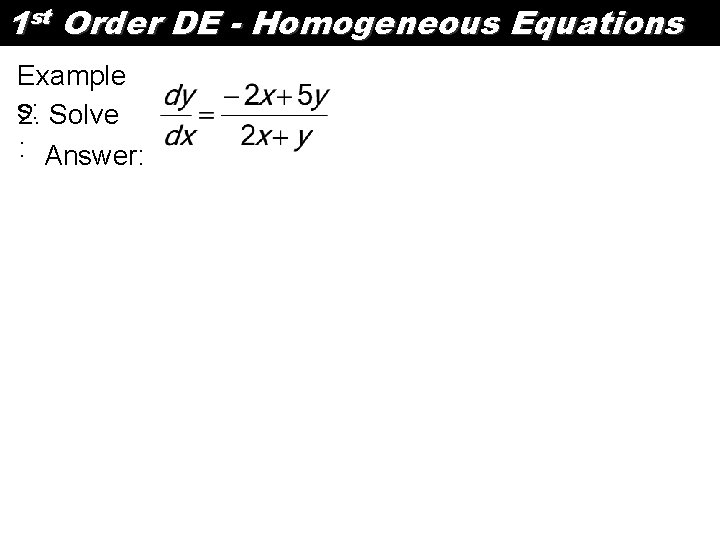1 st Order DE - Homogeneous Equations Example s: 2. Solve : Answer:1 st Order DE – Exact Equation The differential equation M(x, y)dx + N(x, y)dy = 0 is an exact equation if : The solutions are given by the implicit equation where : F/ x = M(x, y) and F/ y = N(x, y) Solution : 1. Integrate either M(x, y) with respect to x or N(x, y) to y. Assume integrating M(x, y), then : 2. Now : or :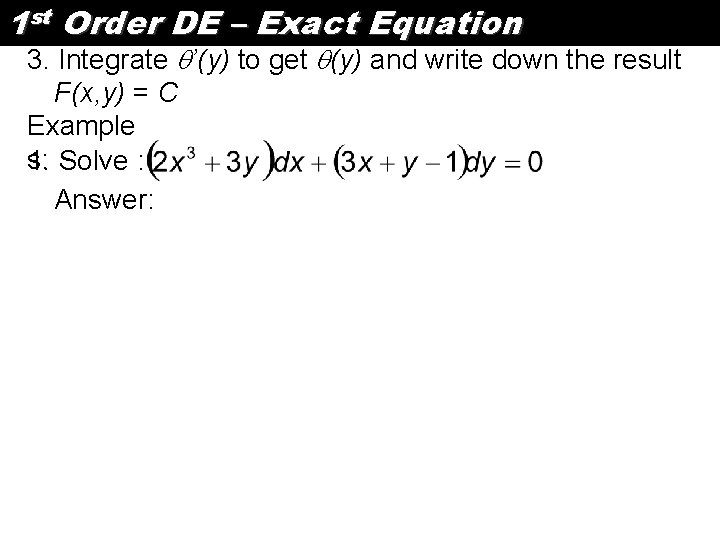1 st Order DE – Exact Equation 3. Integrate ’(y) to get (y) and write down the result F(x, y) = C Example s: 1. Solve : Answer:1 st Order DE – Exact Equation Example s: 2. Solve : Answer:1 st Order DE – Non Exact Equation The differential equation M(x, y)dx + N(x, y)dy = 0 is a non exact equation if : The solutions are given by using integrating factor to change the equation into exact equation Solution : Check if 1. : then integrating factor is or if : then integrating factor is1 st Order DE – Non Exact Equation 2. Multiply the differential equation with integrating factor which result an exact differential equation 3. Solve the equation using procedure for an exact equation1 st Order DE – Non Exact Equation Example s: 1. Solve : Answer: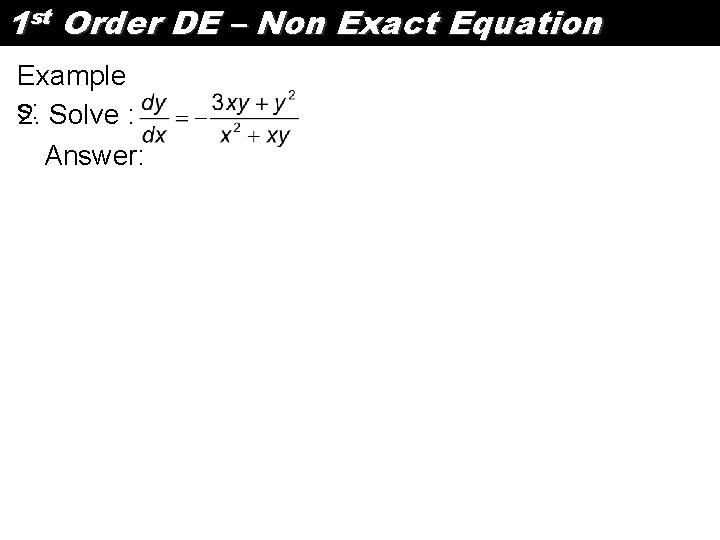1 st Order DE – Non Exact Equation Example s: 2. Solve : Answer:1 st Order DE – Linear Equation A first order linear differential equation has the following general form: Solution : 1. Find the integrating factor: 2. Evaluate : 3. Find the solution: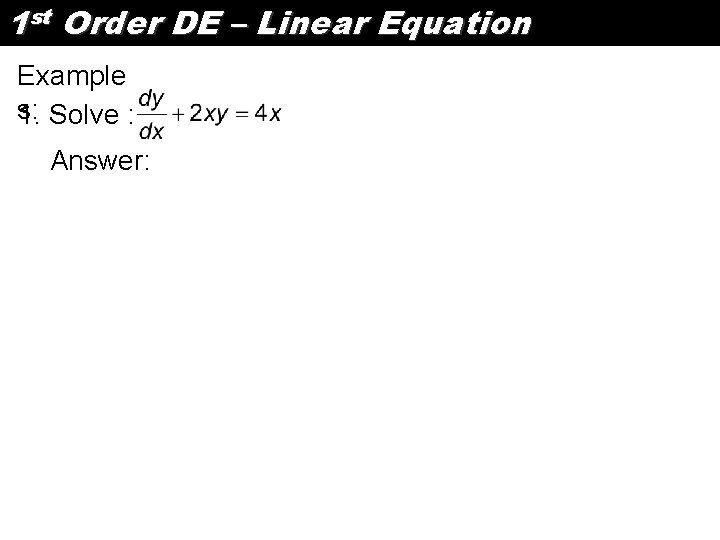1 st Order DE – Linear Equation Example s: 1. Solve : Answer: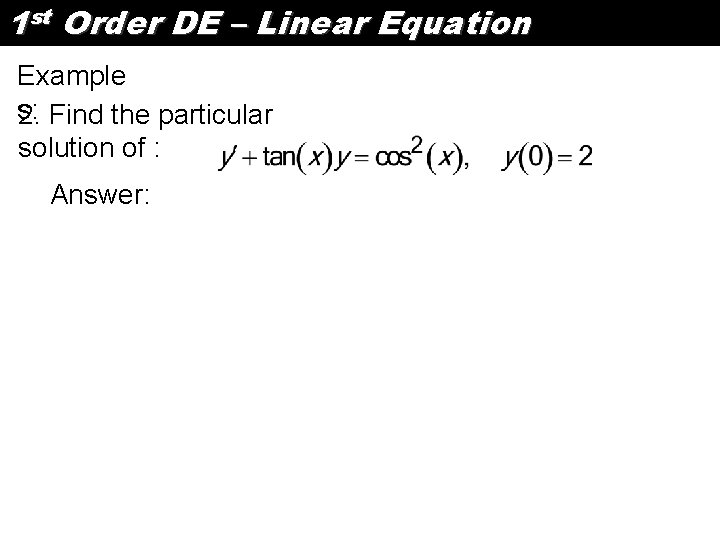1 st Order DE – Linear Equation Example s: 2. Find the particular solution of : Answer: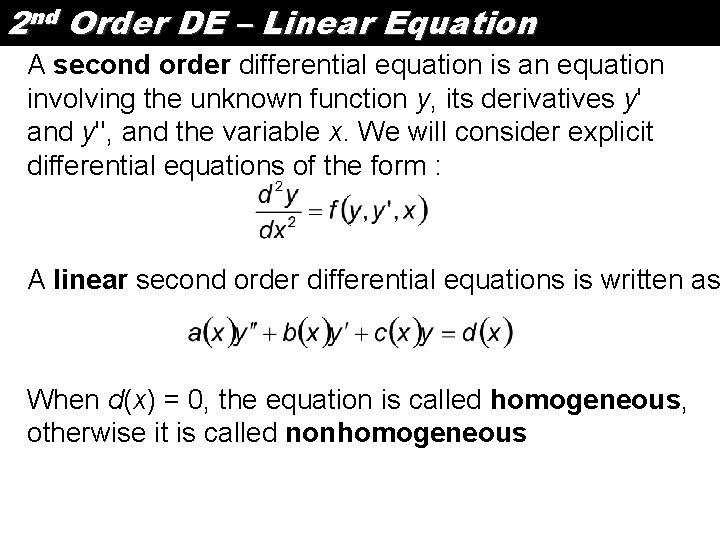2 nd Order DE – Linear Equation A second order differential equation is an equation involving the unknown function y, its derivatives y' and y'', and the variable x. We will consider explicit differential equations of the form : A linear second order differential equations is written as When d(x) = 0, the equation is called homogeneous, otherwise it is called nonhomogeneous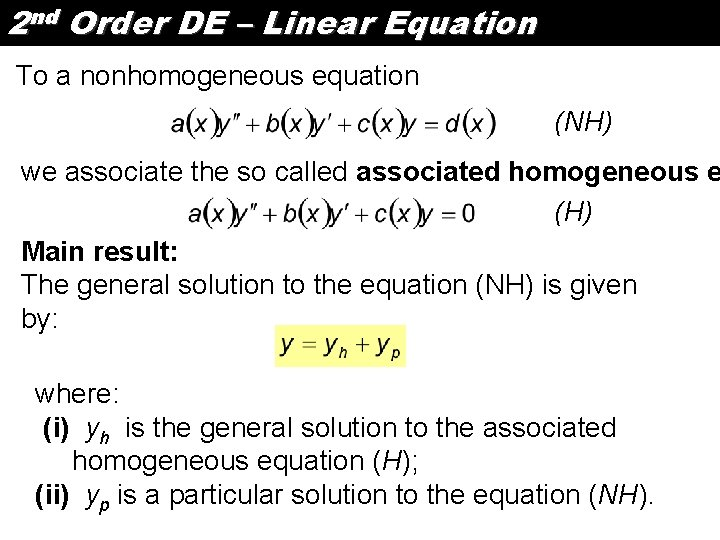2 nd Order DE – Linear Equation To a nonhomogeneous equation (NH) we associate the so called associated homogeneous e (H) Main result: The general solution to the equation (NH) is given by: where: (i) yh is the general solution to the associated homogeneous equation (H); (ii) yp is a particular solution to the equation (NH).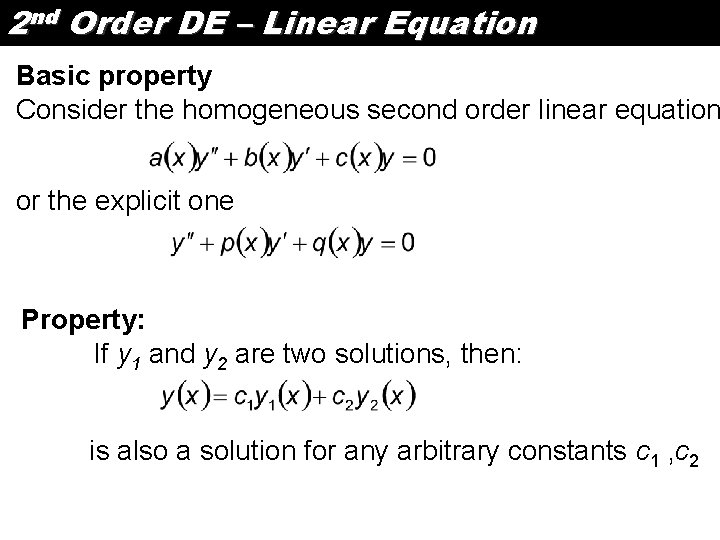2 nd Order DE – Linear Equation Basic property Consider the homogeneous second order linear equation or the explicit one Property: If y 1 and y 2 are two solutions, then: is also a solution for any arbitrary constants c 1 , c 2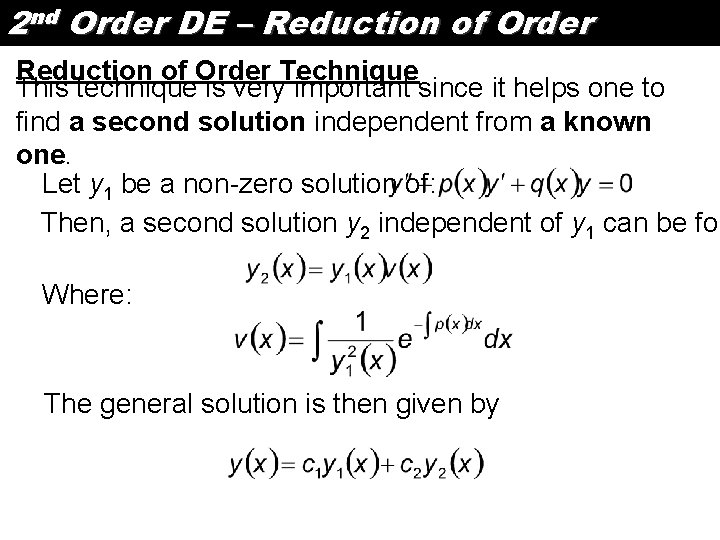2 nd Order DE – Reduction of Order Technique This technique is very important since it helps one to find a second solution independent from a known one. Let y 1 be a non-zero solution of: Then, a second solution y 2 independent of y 1 can be fou Where: The general solution is then given by2 nd Order DE – Reduction of Order Example s: Find the general solution to the Legendre equation 1. Using the fact that : y 1 = x is a solution.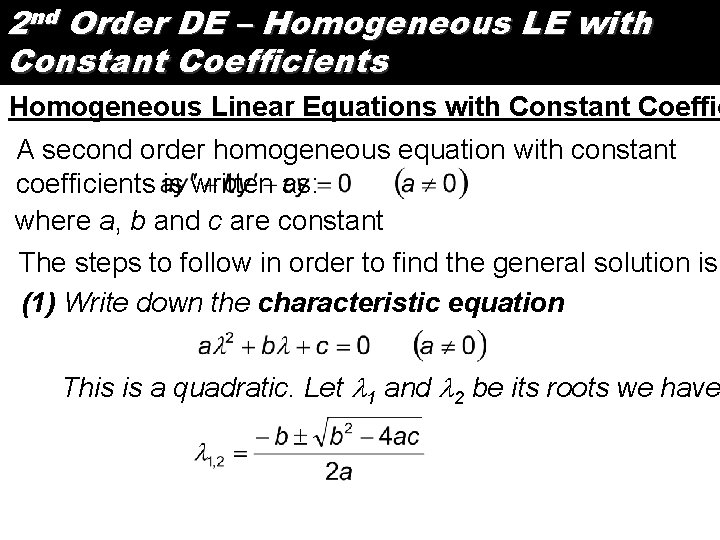2 nd Order DE – Homogeneous LE with Constant Coefficients Homogeneous Linear Equations with Constant Coeffic A second order homogeneous equation with constant coefficients is written as: where a, b and c are constant The steps to follow in order to find the general solution is (1) Write down the characteristic equation This is a quadratic. Let 1 and 2 be its roots we have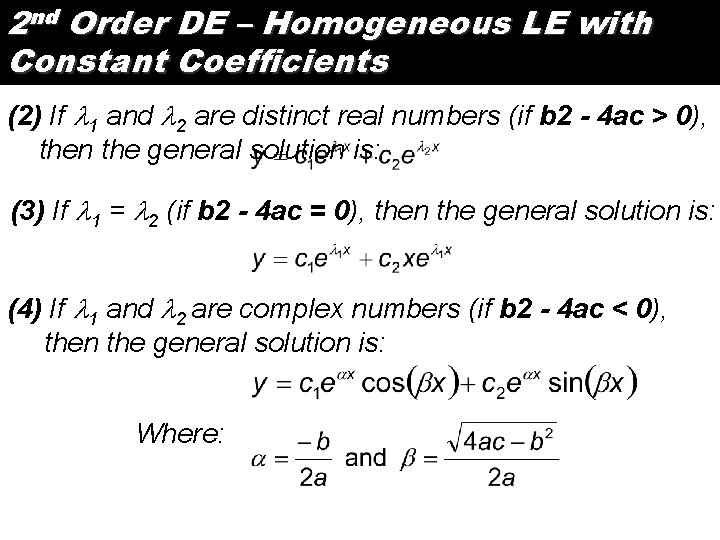2 nd Order DE – Homogeneous LE with Constant Coefficients (2) If 1 and 2 are distinct real numbers (if b 2 - 4 ac > 0), then the general solution is: (3) If 1 = 2 (if b 2 - 4 ac = 0), then the general solution is: (4) If 1 and 2 are complex numbers (if b 2 - 4 ac < 0), then the general solution is: Where:2 nd Order DE – Homogeneous LE with Constant Coefficients 1. Find the solution to the Initial Value Problem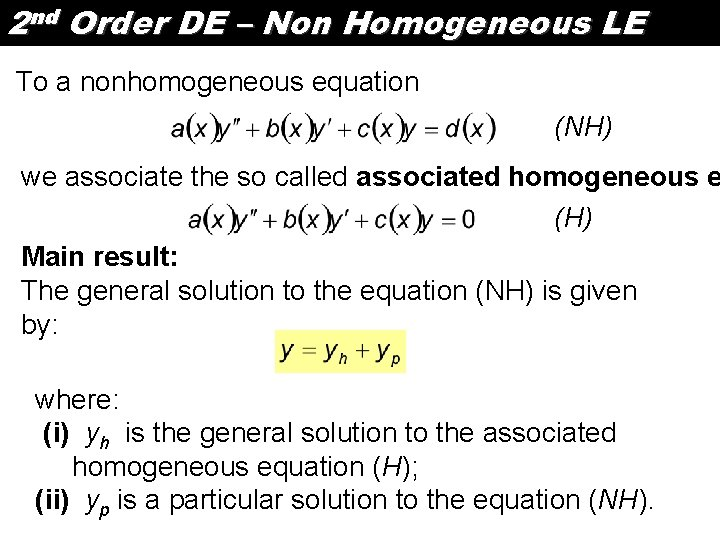2 nd Order DE – Non Homogeneous LE To a nonhomogeneous equation (NH) we associate the so called associated homogeneous e (H) Main result: The general solution to the equation (NH) is given by: where: (i) yh is the general solution to the associated homogeneous equation (H); (ii) yp is a particular solution to the equation (NH).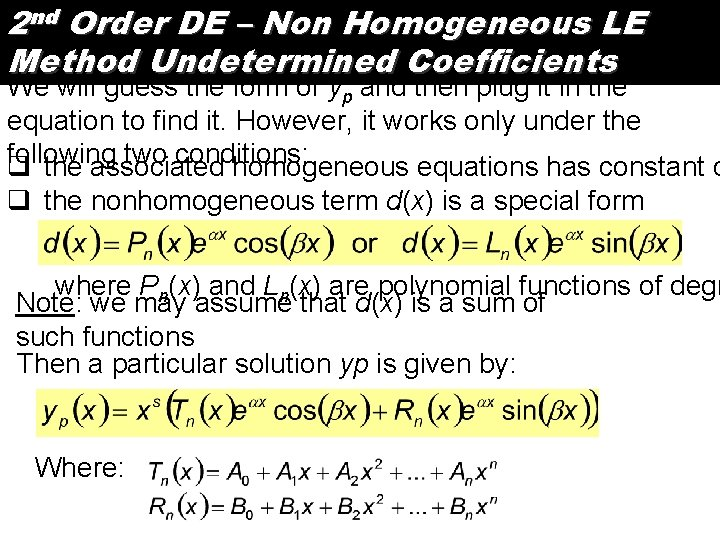2 nd Order DE – Non Homogeneous LE Method Undetermined Coefficients We will guess the form of yp and then plug it in the equation to find it. However, it works only under the following two conditions: q the associated homogeneous equations has constant c q the nonhomogeneous term d(x) is a special form where Pn(x) and Ln(x) are polynomial functions of degr Note: we may assume that d(x) is a sum of such functions Then a particular solution yp is given by: Where:2 nd Order DE – Non Homogeneous LE Method Undetermined Coefficients The steps to follow in applying this method: 1. Check that the two conditions are satisfied 2. Write down the characteristic equation and find its root 3. Write down the number 4. Compare this number to the roots of the characteristic If : 5. Write down the form of particular solution Where: 6. Find constant A and B by plugging yp solution to origin2 nd Order DE – Non Homogeneous LE Method Undetermined Coefficients 1. Find a particular solution to the equation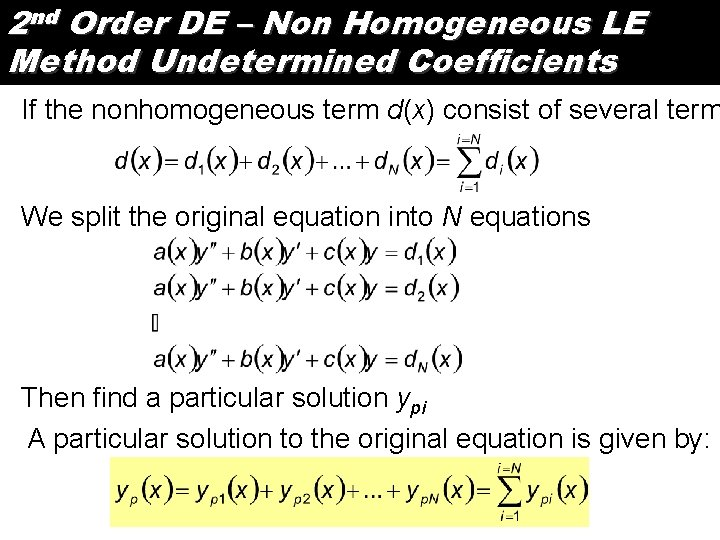2 nd Order DE – Non Homogeneous LE Method Undetermined Coefficients If the nonhomogeneous term d(x) consist of several term We split the original equation into N equations Then find a particular solution ypi A particular solution to the original equation is given by:2 nd Order DE – Non Homogeneous LE Method Undetermined Coefficients If the nonhomogeneous term d(x) consist of several term We split the original equation into N equations Then find a particular solution ypi A particular solution to the original equation is given by:2 nd Order DE – Non Homogeneous LE Method Undetermined Coefficients 1. Find a particular solution to the equation